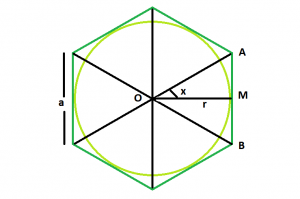# Area of a circle inscribed in a regular hexagon

Given a regular Hexagon with side length a, the task is to find the area of the circle inscribed in it, given that, the circle is tangent to each of the six sides.

Examples:

```Input: a = 4
Output: 37.68

Input: a = 10
Output: 235.5
```

## Recommended: Please try your approach on {IDE} first, before moving on to the solution.Approach:
From the figure, it is clear that, we can divide the regular hexagon into 6 identical equilateral triangles.
We take one triangle OAB, with O as the centre of the hexagon or circle, & AB as one side of the hexagon.
Let M be mid-point of AB, OM would be the perpendicular bisector of AB, angle AOM = 30 deg

Then in right angled triangle OAM,

tanx = tan30 = 1/√3
So, a/2r = 1/√3
Therefore, r = a√3/2
Area of circle, A =Πr²=Π3a^2/4

Below is the implementation of the approach:

## C++

 `// C++ Program to find the area of the circle ` `// which can be inscribed within the hexagon ` `#include ` `using` `namespace` `std; ` ` `  `// Function to find the area ` `// of the inscribed circle ` `float` `circlearea(``float` `a) ` `{ ` ` `  `    ``// the side cannot be negative ` `    ``if` `(a < 0) ` `        ``return` `-1; ` ` `  `    ``// area of the circle ` `    ``float` `A = (3.14 * 3 * ``pow``(a, 2)) / 4; ` ` `  `    ``return` `A; ` `} ` ` `  `// Driver code ` `int` `main() ` `{ ` `    ``float` `a = 4; ` `    ``cout << circlearea(a) << endl; ` ` `  `    ``return` `0; ` `} `

## Java

 `//Java program to find the  ` `//area of the circle  ` `//which can be inscribed within the hexagon ` ` `  `import` `java.util.*; ` ` `  `class` `solution ` `{ ` `static` `double` `circlearea(``double` `a) ` `{ ` ` `  `// the side cannot be negative ` `    ``if` `(a < ``0``) ` `    ``return` `-``1``; ` ` `  `// area of the circle ` `    ``double` `A = (``3.14` `* ``3` `* Math.pow(a,``2``) ) / ``4``; ` ` `  `    ``return` `A; ` `} ` `public` `static` `void` `main(String arr[]) ` `{ ` `    ``double` `a = ``4``; ` `    ``System.out.println(circlearea(a)); ` `} ` `} `

## Python 3

 `# Python 3 program to find the  ` `# area of the circle  ` `# which can be inscribed within the hexagon ` ` `  `# Function to find the area  ` `# of the inscribed circle  ` `def` `circlearea(a) : ` ` `  `    ``# the side cannot be negative  ` `    ``if` `a < ``0` `: ` `        ``return` `-``1` ` `  `    ``#  area of the circle ` `    ``A ``=` `(``3.14` `*` `3` `*` `pow``(a,``2``)) ``/` `4` ` `  `    ``return` `A ` ` `  ` `  `# Driver code      ` `if` `__name__ ``=``=` `"__main__"` `: ` ` `  `    ``a ``=` `4` `    ``print``(circlearea(a)) ` ` `  ` `  `# This code is contributed by ANKITRAI1 `

## C#

 `// C# program to find   ` `// the area of the circle  ` `// which can be inscribed  ` `// within the hexagon ` `using` `System; ` ` `  `class` `GFG ` `{ ` `static` `double` `circlearea(``double` `a) ` `{ ` ` `  `    ``// the side cannot be negative ` `    ``if` `(a < 0) ` `    ``return` `-1; ` ` `  `    ``// area of the circle ` `    ``double` `A = (3.14 * 3 *  ` `                ``Math.Pow(a, 2)) / 4; ` ` `  `    ``return` `A; ` `} ` ` `  `// Driver Code ` `public` `static` `void` `Main() ` `{ ` `    ``double` `a = 4; ` `    ``Console.WriteLine(circlearea(a)); ` `} ` `} ` ` `  `// This code is contributed ` `// by inder_verma `

## PHP

 `

Output:

```37.68
```

Attention reader! Don’t stop learning now. Get hold of all the important DSA concepts with the DSA Self Paced Course at a student-friendly price and become industry ready.

My Personal Notes arrow_drop_upCheck out this Author's contributed articles.

If you like GeeksforGeeks and would like to contribute, you can also write an article using contribute.geeksforgeeks.org or mail your article to contribute@geeksforgeeks.org. See your article appearing on the GeeksforGeeks main page and help other Geeks.

Please Improve this article if you find anything incorrect by clicking on the "Improve Article" button below.